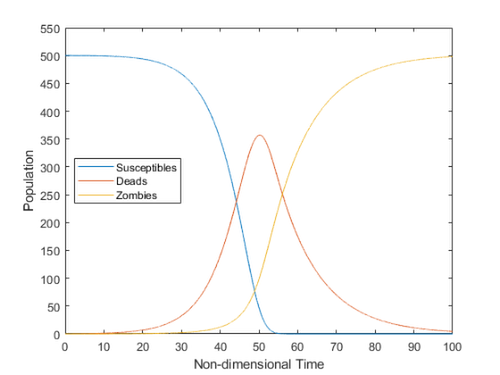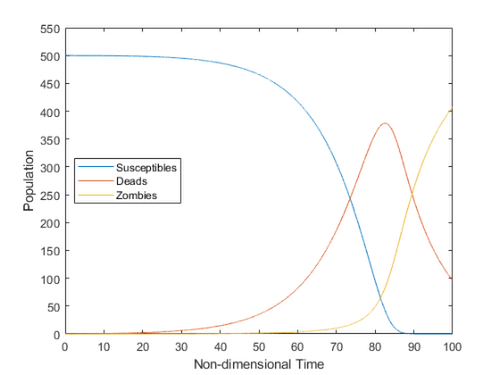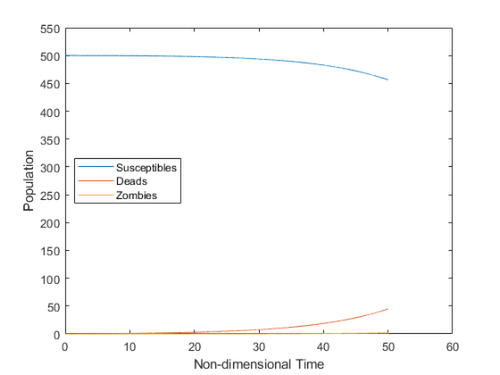# Zombie Outbreak

Sean‘s pick this week is Mathematical modelling of an outbreak of zombie infection by E. Cheynet.

In honor of Halloween, E. has provided us with a way to simulate zombie outbreaks! Do you have what it takes to save humanity?

Npop = 500; % population
alpha = 7.5e-3 ; %  "zombie destruction" rate.
beta = 5e-3; % "zombie creation" rate.
zeta = 9.2e-2; % zombie resurrection rate
delta = 1.2e-4; % susceptibles death rate
tmax = 100; % max duration of the simulation (non-dimensional)
dt = 0.005; % time step

[SRZ, t] = zombies(Npop, alpha, beta, zeta, delta, tmax, dt, 0);
plotPopulations(t, SRZ, Npop)
snapnowHmm, what if we work on our sharp shooter skills and eat healthy?

alpha = 1e-2; % Go to the range
delta = 9e-5; % Add broccoli
[SRZ, t] = zombies(Npop, alpha, beta, zeta, delta, tmax, dt, 0);
plotPopulations(t, SRZ, Npop)
snapnowThat only delays the inevitable; skip the broccoli, we need to execute a mass extermination of zombies. The erad function gives us the ability to raid them.

delta = 1.2e-4; % Back to pizza
k = 0.65;
Nraid = 4;
[SRZ, t] = erad(Npop, alpha, beta, zeta, delta, k, Nraid, tmax, dt,0);
plotPopulations(t, SRZ, Npop)
Zombies have been eradicated (until now...).Unfortunately, the model doesn’t seem to account for new humans being born, so I think any simulation eventually ends with us all dead… Have a great weekend!

Give it a try and let us know what you think here or leave a comment for E.

function plotPopulations(t, SRZ, Npop)
figure
plot(t, SRZ);
ylim([0, 1.1*Npop])